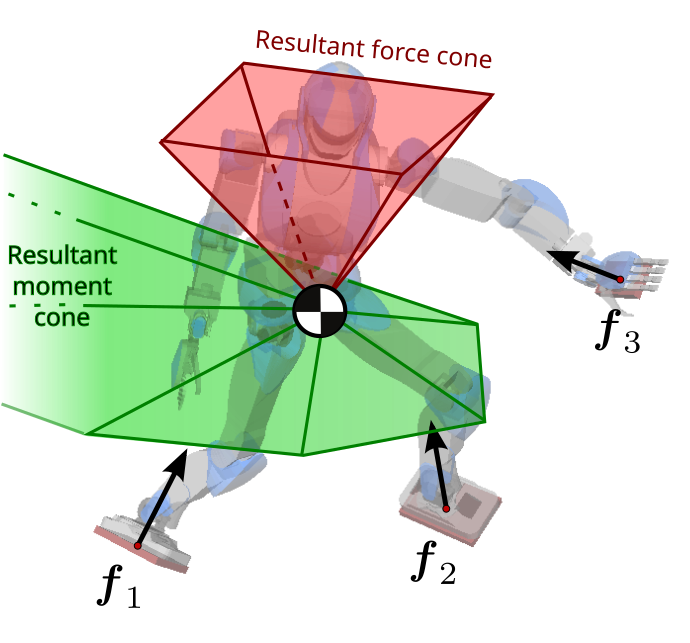# Wrench friction cones

Wrench friction cones are 6D polyhedral convex cones that characterize feasible contact wrenches, that is to say, wrenches that can be realized during contact-stable motions. They generalize Coulomb friction cones to rigid body motions, and can also be used to encode other limitations such as maximum joint torques.

## Contact Wrench ConeThe contact wrench cone (CWC) is the friction cone of the (net) contact wrench acting on the robot in multi-contact, i.e. the sum of all contact wrenches. It can be computed analytically in simple cases, or using numerical polyhedral projection algorithms.

By construction, a solution can be found to the contact force optimization QP if and only if the centroidal momentum $$(\bfpdd_G, \dot{\bfL}_G)$$ lies in the CWC. When there is a large number of momenta to check, it is computationally more efficient to compute the CWC as a matrix $$\bfU$$ first, and then perform each check as $$\bfU [\bfpdd_G\ \dot{\bfL}_G] \leq \boldsymbol{0}$$ rather than solving a large number of quadratic programs.

On an advanced note: in the figure above, the CWC is represented by a red force cone and a green moment cone. This is a drawing convenience: in practice, the CWC is a 6D cone where force and moment are not independent. If you choose a resultant force in the red cone, it will affect the shape of the green one. And conversely, fixing the resultant moment to a given value (e.g. zero, see below) will affect the shape of the red cone.

## Contact-stability areas and volumes

When additional constraints are imposed on the centroidal motion, the 6D centroidal wrench cone reduces to lower-dimensional areas and volumes that can be used for planning or control:

• When the robot is not moving, contact stability is characterized by the static equilibrium polygon: the configuration of the robot is feasible (sustainable) if and only if the center of mass lies in a specific polygon, which can be computed efficiently.

• When the robot moves in the Linear Inverted Pendulum Mode (LIPM, i.e. with conserved angular momentum and keeping the center of mass in a plane), the CWC reduces to a ZMP support area.

• When the robot moves with a conserved angular momentum $$(\Ld_G = 0)$$, the CWC reduces to a 3D cone over center-of-mass accelerations that can be used e.g. for multi-contact locomotion.

## To go further

Stability conditions are used extensively in motion generation, for instance in multi-contact walking pattern generation. A description of the computation of the CWC can be found in this paper. The algorithms themselves are implemented in:

• The ContactSet class of the pymanoid library (Python).
• wrench-cone-lib: a C++ library with Python bindings.

Wrench friction cones can represent all sorts of power limitations, including but not restricted to friction. One example is given by the torque-limited wrench cones used in this predictive controller for impact absorption, which can be projected into actuation-aware center-of-mass support areas.

Pages of this website are under the CC-BY 4.0 license.